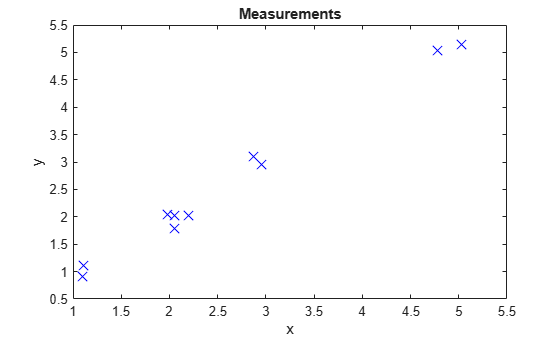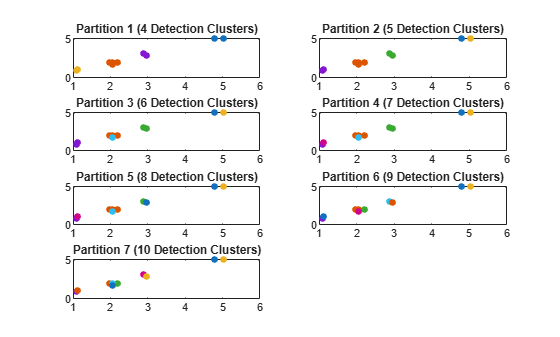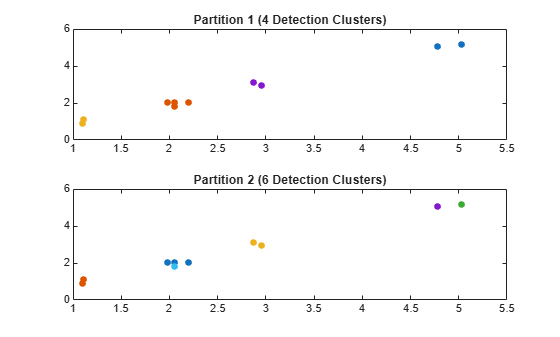partitionDetections

Partition detections based on distance

Description

A partition of a set of detections is defined as a division of these detections into nonempty mutually exclusive detection cells. Using multiple distance thresholds, you can use the function to separate detections into different detection cells and get all the possible partitions using either distance-partitioning or density-based spatial clustering of applications with noise (DBSCAN). Additionally, you can choose the distance metric as Mahalanobis distance or Euclidean distance by specifying the 'Distance' Name-Value pair argument.

Distance Partitioning

Distance partitioning is the default partitioning algorithm of partitionDetections. In distance partitioning, a detection cluster comprises of detections whose distance to at least one other detection in the cluster is less than the distance threshold. In other words, two detections belong to the same detection cluster if their distance is less than the distance threshold. To use distance-partitioning, you can specify the 'Algorithm' Name-Value argument as 'Distance-Partitioning' or simply do not specify the 'Algorithm' argument.

example

partitions = partitionDetections(detections) returns possible partitions for detections using the distance-partitioning algorithm. By default, the function uses the distance partitioning algorithm and considers all real value Mahalanobis distance thresholds between 0.5 and 6.25 and returns a maximum of 100 partitions.

partitions = partitionDetections(detections,tLower,tUpper) specifies the lower and upper bounds of the distance thresholds, tLower and tUpper.

partitions = partitionDetections(detections,tLower,tUpper,'MaxNumPartitions',maxNumber) specifies the maximum number of allowed partitions, maxNumber.

partitions = partitionDetections(detections,allThresholds)specifies the exact thresholds considered for partition.

[partitions,indexDP] = partitionDetections(detections,allThresholds) additionally returns an index vector indexDP representing the correspondence between all thresholds and the resulting partitions.

DBSCAN Partitioning

To use the DBSCAN partitioning, specify the 'Algorithm' argument as 'DBSCAN'.

partitions = partitionDetections(detections,'Algorithm','DBSCAN') returns possible partitions of the detections by using DBSCAN partitioning and ten distance threshold (epsilon or neighbor search radius) values linearly spaced between 0.25 and 6.25. By default, each cluster must contain at least three points.

example

partitions = partitionDetections(detections,epsilon,minNumPoints,'Algorithm','DBSCAN') specifies the distance thresholds epsilon and the minimum number of points per cluster minNumPoints of the DBSCAN algorithm.

[partitions,indexDB] = partitionDetections(detections,epsilon,minNumPoints,'Algorithm','DBSCAN') additionally returns an index vector indexDB representing the correspondence between the threshold values epsilon and the resulting partitions.

Specify Distance Metric

Using the 'Distance' Name-Value argument, you can specify the distance metric used in the partitioning.

___ = partitionDetections(___,'Distance',distance) additionally specifies the distance metric as 'Mahalanobis' or 'Euclidean'. Use this syntax with any of the input or output arguments in previous syntaxes.

Examples

collapse all

Generate 2-D detections using objectDetection.

rng(2018); % For reproducible results
detections = cell(10,1);
for i = 1:numel(detections)
id = randi([1 5]);
detections{i} = objectDetection(0,[id;id] + 0.1*randn(2,1));
detections{i}.MeasurementNoise = 0.01*eye(2);
end

Extract and display generated position measurements.

d = [detections{:}];
measurements = [d.Measurement];

figure()
plot(measurements(1,:),measurements(2,:),'x','MarkerSize',10,'MarkerEdgeColor','b')
title('Measurements')
xlabel('x')
ylabel('y')Generate partitions from the detections using distance partitioning and count the number of partitions.

partitions = partitionDetections(detections);
numPartitions = size(partitions,2);

Visualize the partitions. Each color represents a detection cluster.

figure()
for i = 1:numPartitions
numCells = max(partitions(:,i));
subplot(4,ceil(numPartitions/4),i);
for k = 1:numCells
ids = partitions(:,i) == k;
plot(measurements(1,ids),measurements(2,ids),'.','MarkerSize',15);
hold on;
end
title(['Partition ',num2str(i),' (',num2str(k),' Detection Clusters)']);
endGenerate 2-D detections using objectDetection.

rng(2018); % For reproducible results
detections = cell(10,1);
for i = 1:numel(detections)
id = randi([1 5]);
detections{i} = objectDetection(0,[id;id] + 0.1*randn(2,1));
detections{i}.MeasurementNoise = 0.01*eye(2);
end

Extract and display generated position measurements.

d = [detections{:}];
measurements = [d.Measurement];

figure()
plot(measurements(1,:),measurements(2,:),'x','MarkerSize',10,'MarkerEdgeColor','b')
title('Measurements')
xlabel('x')
ylabel('y')Generate partitions from the detections using DBSCAN and count the number of partitions.

[partitions,index] = partitionDetections(detections,[1.6;2],2,'Algorithm','DBSCAN');
numPartitions = size(partitions,2);

Visualize the partitions. Each color represents a detection cluster.

figure()
for i = 1:numPartitions
numCells = max(partitions(:,i));
subplot(2,ceil(numPartitions/2),i);
for k = 1:numCells
ids = partitions(:,i) == k;
plot(measurements(1,ids),measurements(2,ids),'.','MarkerSize',15);
hold on;
end
title(['Partition ',num2str(i),' (',num2str(k),' Detection Clusters)']);
endFrom the index values, the first partition corresponds to an epsilon value of 2 and the second partition corresponds to an epsilon value of 1.6.

index
index = 1×2 uint32 row vector

2   1

Input Arguments

collapse all

Object detections, specified as an N-element array of objectDetection objects, an N-element cell array of objectDetection objects, or an N-element array of structures whose field names are the same as the property names of the objectDetection object, where N is the number of detections. You can create detections directly, or you can obtain detections from the outputs of sensor objects, such as fusionRadarSensor, irSensor, and sonarSensor.

Data Types: cell

Distance metric for partitioning, specified as 'Mahalanobis' or 'Euclidean'.

Distance Partitioning

Lower bound of the distance thresholds, specified as a scalar. This argument sets the lower bound of the distance thresholds considered for distance partitioning.

Example: 0.05

Data Types: double

Upper bound of the distance thresholds, specified as a scalar. This argument sets the upper bound of the distance thresholds considered for distance partitioning.

Example: 0.98

Data Types: double

Maximum number of allowed partitions for distance partitioning, specified as a positive integer.

Example: 20

Data Types: double

All thresholds for distance partitioning, specified as an M-element real-valued vector. The function calculates partitions based on each threshold value provided in allThresholds. Note that multiple thresholds can result in the same partition, and the function output partitions, returned as an N-by-Q matrix with QM, contains only unique partitions.

Example: [0.1;0.2;0.35;0.4]

Data Types: double

DBSCAN

All thresholds for DBSCAN, specified as an M-element real-valued element vector. The function calculates partitions based on each threshold value provided in epsilon. Note that multiple thresholds can result in the same partition, and the function output partitions, returned as an N-by-Q matrix with QM, contains only unique partitions.

Example: [0.1;0.2;0.35;0.4]

Data Types: double

Minimum number of points for each cluster in the partition, specified as a positive integer that applies to all epsilon values or as an M-element vector of positive integers, where M is the length of the epsilon vector.

Example: 20

Data Types: double

Output Arguments

collapse all

Partitions of detections, returned as an N-by-Q matrix. N is the number of detections and Q is the number of partitions. Each column of the matrix represents a valid partition. In each column, the value of the ith element represents the identity number of the cluster that the ith detection belongs to. For example, given a partition matrix P, if P(i,j) = k, then in partition j, detection i belongs to cluster k.

Index vector for distance partitioning, returned as an M-element vector of positive integers. Each element of the index vector is a partition index yielded by the corresponding threshold value in the allThreshould input argument. For example, if indexDP(i) = k, then allThresholds(i) corresponds to the partition specified by partitions(:,k).

Data Types: double

Index vector for DBSCAN, returned as an M-element vector of positive integers. Each element of the index vector is a partition index yielded by the corresponding threshold value in the epsilon input argument. For example, if indexDB(i) is k, then epsilon(i) corresponds to the partition specified by partitions(:,k).

Data Types: double

 Granstrom, Karl, Christian Lundquist, and Omut Orguner. “Extended Target Tracking Using a Gaussian-Mixture PHD Filter.” IEEE Transactions on Aerospace and Electronic Systems 48, no. 4 (October 2012): 3268–86. https://doi.org/10.1109/TAES.2012.6324703.

 Ester, Martin, Hans-Peter Kriegel, Jörg Sander, and Xiaowei Xu. “A Density-Based Algorithm for Discovering Clusters in Large Spatial Databases with Noise.” In Proceedings of the Second International Conference on Knowledge Discovery and Data Mining, 226–31. KDD’96. Portland, Oregon: AAAI Press, 1996.